# Luggage and air travel

Two friends traveling by plane had a total of 35 kg of luggage. They paid one 72 CZK and second 108 CZK for being overweight. If only one paid for all the bags, it would cost 300 CZK. What weight of baggage did each of them have, how many kilograms of luggage could a single passenger carry for free?

a =  16 kg
b =  19 kg
x =  10 kg

### Step-by-step explanation:

a+b = 35
a-x = 72p
b-x = 108p
a+b-x = 300p

a+b = 35
a-x = 72•p
b-x = 108•p
a+b-x = 300•p

a+b = 35
a-72p-x = 0
b-108p-x = 0
a+b-300p-x = 0

a = 16
b = 19
p = 1/12 ≈ 0.083333
x = 10

Our linear equations calculator calculates it.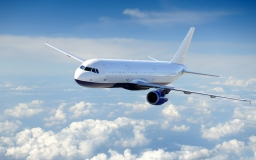We will be pleased if You send us any improvements to this math problem. Thank you!Tips to related online calculators
Do you have a system of equations and looking for calculator system of linear equations?

## Related math problems and questions:

• Two teas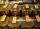A mixture weighing 10 kg was made from two types of tea. The price of tea was 160 CZK/kg and second tea 170 CZK/kg. The price of the mixture is 166 CZK/kg. How many kilograms of each type of tea had to be mixed?
• Coffee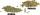Coffee merchant has coffee robusta and arabica species. 1 kg Robusta worth 450 CZK, Arabica 1 kg is 300 CZK more expensive. Calculate how many kilograms of Robusta and Arabica will need to produce 30 kg of the mixture so that the mixture cost is 490 CZK p
• Mixture of coffee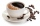They have to prepare a mixture of coffee in packing centres so that 1 kg costs 240 CZK. There are two types of coffee in stock at the price of 220 CZK per 1 kg and 300 CZK per 1 kg. How many kilograms of each species need to be mixed to prepare 50 kg of t
• Purchase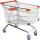Mother bought 5 boxes of milk and 7 kg of potatoes and paid a total CZK 147. Aunt bought 7 boxes of milk and 3 kg of potatoes and paid 131 CZK. What is the price of one carton of milk and 1 kg of potatoes? How CZK together would have saved if bought at th
• Bonbons 2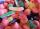Kilo sweets will cost 260 CZK. The first type has a price per 320 kg, the second type 240 CZK per kg. How many kilos of both kinds of sweets need to prepare a 35 kg mixture ?
• Candies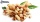In the confectionery, the price for 1 kg of pistachio candies cost CZK 360, and the price for 1 kg of hazelnut candies was CZK 280. Mixing these two types of sweets created a box of chocolates. How many grams of pistachios and how many grams of hazelnut c
• Apples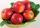Zuzana bought 3 kg Jonathan apples. Then she noticed that Golden apples were 3 CZK a kilogram cheaper. So they bought 2 kg. For apples, she paid a total of CZK 109. How much did 1 kg Jonathan and how much Golden?
• Coffee shop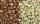To the coffee shop brought 2 types of coffee totally 50 kg. The first type was CZK 220 per kilogram, coffee second type 300 CZK per 1 kg. For all the coffee trader earned CZK 12,000. How many kilograms of coffee of first type and how many kilograms of cof
• CoffeeOne kilogram of cheaper coffee costs CZK 150, one kilogram of more expensive coffee costs CZK 200. We have to prepare a mixture of 35 kg of coffee for 180 CZK. How do we prepare the mixture (how many of which kind of coffee do we need)?
• SweetsWe want to prepare 5 kg of sweets for 150 CZK. We will mix cheaper candy: 1 kg for 120 CZK and more expensive candy: 1 kg per 240 CZK. How much of these two types of candy is necessary to prepare this mixture?
• Bananas and mango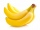5 kg of bananas and three kilograms of mango cost 146 CZK, two kilograms of bananas and 5 kilograms of mango cost 142 CZK. How much is a kilogram of bananas, and how many mangoes?
• Barrel 3Barrel with water has a weight 118 kg. When we get off 75% of water it has a weight 35 kg. How many kg has empty barrel?
• CandyThe price for 1kg of more expensive candy is 125 CZK. The price for 1kg of cheaper candy is 100 CZK. We make two different blends of candy. And now. The second blend contains 2kg of more expensive candy and several kg of cheaper candy. The price per 1 kg
• Tea mixture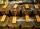Of the two sort of tea at a price of 180 CZK/kg and 240 CZK/kg we make a mixture 12 kg that should be prepared at a price of 200 CZK / kg. How many kilos of each sort of tea will we need to be mixed?
• CandiesThe price for 1 kg of more expensive candies is 125 CZK. The price for 1 kg of cheaper candy is 100 CZK. We mix two different mixtures of candy. A) The first mixture contains 2 kg more expensive and 0.5 kg cheaper candy. Calculate the price for 1 kg of th
• Three piles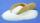100 kg of sugar we divide into three piles. The first pile is small. If we added to second 2 kg of sugar it would have 25% more sugar than the first pile. If we added to third pile 3 kg of sugar it would have 20% more sugar than the 2nd pile. How many kil
• The water barrel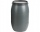The water barrel weighs 122 kg. If we pour 75% of the water out of it, it will weigh 35 kg. What is the weight of the barrel?